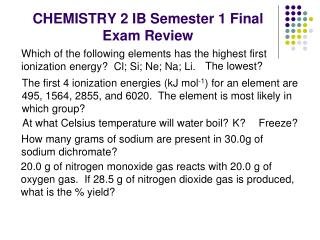# CHEMISTRY 2 IB Semester 1 Final Exam Review - PowerPoint PPT PresentationDownload PresentationCHEMISTRY 2 IB Semester 1 Final Exam Review

CHEMISTRY 2 IB Semester 1 Final Exam Review
Download Presentation## CHEMISTRY 2 IB Semester 1 Final Exam Review

- - - - - - - - - - - - - - - - - - - - - - - - - - - E N D - - - - - - - - - - - - - - - - - - - - - - - - - - -
##### Presentation Transcript

1. CHEMISTRY 2 IB Semester 1 Final Exam Review Which of the following elements has the highest first ionization energy? Cl; Si; Ne; Na; Li. The lowest? The first 4 ionization energies (kJ mol-1) for an element are 495, 1564, 2855, and 6020. The element is most likely in which group? At what Celsius temperature will water boil? K? Freeze? How many grams of sodium are present in 30.0g of sodium dichromate? 20.0 g of nitrogen monoxide gas reacts with 20.0 g of oxygen gas. If 28.5 g of nitrogen dioxide gas is produced, what is the % yield?

2. The emission spectrum of hydrogen consists of several series of sharp emission lines in the ultraviolet (Lyman series) in the visible (Balmer series) and in the infrared (Paschen series, Brackett series, etc.) regions of the spectrum. (a) What feature of the electronic energies of the hydrogen atom explains why the emission spectrum consists of discrete wavelengths rather than a continuum of wavelengths? (b) Account for the existence of several series of lines in the spectrum. What quantity distinguishes one series of lines from another? What is the difference b/w random and systematic error? Give an example for each.

3. How much water must be evaporated from 500. mL of 1.00M NaOH to make it 2.50M? A 0.500 Msolution could be produced by dissolving how many g of sodium hydroxide in enough water to make 250. mL of solution? What is the mass of ethane gas that contains 1.50 x 1023 hydrogen atoms? What is the molecular formula of a compound composed of 25.9% N and the rest O. Mr = 108.02 Determine whether each of the following compounds is ionic or covalent: CH4, BaF2, N2O5, PCl5 Choose 3 forms to express electron structure for S, Cl-, Fe3+.

4. The molecular formula of a hydrocarbon is to be determined by analyzing its combustion products and investigating its colligative properties. The hydrocarbon burns completely, producing 7.2 grams of water and 14.1 g of CO2 at standard conditions. What is the empirical formula of the hydrocarbon? Determine the molecular formula if the Mr of the hydrocarbon is 58.14 g/mol. What’s it called? (c) Calculate the mass in grams of O2 required for the complete combustion of the sample of the hydrocarbon described in (a). List, in order, the stages of operation for a mass spectrometer. What information does it provide?

5. Determine the number of protons, neutrons, and electrons in each of the following: 24Mg2+; 50Cr6+ For each of the following, draw the Lewis structure. Predict the shape, bond angle, hybridization and polarity: BH3, SF4, GeCl3- Rank the following in order of increasing water solubility: C2H6, C2H5OH, C12H25OH Write the molecular, complete ionic and net ionic equations for the reaction that would occur between aqueous potassium carbonate and aqueous mercury(I) nitrate. In which of the following quantum energy level jumps would an electron emit a photon with the largest wavelength? n = 4 → n = 2; n = 3 → n = 2; n = 4 → n = 3; n = 2 → n = 4

6. The freezing point and electrical conductivities of three aqueous solutions are given below. Solution Freezing Electrical (0.010 molal) Point Conductivity sucrose -0.0186°C almost zero formic acid -0.0213°C low sodium formate -0.0361°C high Explain the relationship between the freezing point and electrical conductivity for each of the solutions above. Account for the differences in the freezing points among the three solutions.

7. Propane, C3H8, is a hydrocarbon that is commonly used as fuel for cooking. (a) Write a balanced equation for the complete combustion of propane gas, which yields CO2(g) and H2O(l). (b) The heat of combustion of propane is -2,220.1 kJ/mol. Calculate the heat of formation, ΔHf°, of propane given that ΔHf° of H2O(l) = -285.3 kJ/mol and ΔHf° of CO2(g) = -393.5 kJ/mol. (c) Assuming that all of the heat evolved in burning 30.0 grams of propane is transferred to 8.00 kilograms of water (specific heat = 4.18 J/g.K), calculate the increase in temperature of water.

8. Using the equations below: Cu(s) + ½ O2(g) CuO(s) H0 = -156 kJ 2Cu(s) + ½ O2(s)  Cu2O(s) H0 = -170 kJ what is the value of H0 (in kJ) for the following reaction? 2CuO(s)  Cu2O(s) + ½ O2(g)